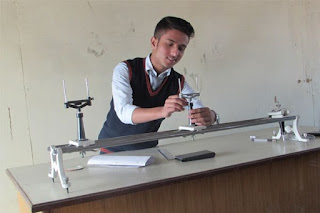# CBSE Class 12 Physics Lab Manual Work Practical Syllabus

This is the syllabus of Physics Lab Works. Hope you will understand it .Keep Reading 😊.

List of Experiments to be performed for Class 12 during practical exams in Physics subject. The list of experiments include the practicals from Physics and truly based NCERT Physics Laboratory Manual books. Click on the list to get the details of the experiments for lab manual works.

These laboratory manual works are prescribed by the CBSE for Class 11 Science students. Get the details to complete your Physics lab manual practical works which is based on NCERT.

Physics Practical WorksSection A

#### Experiments

Experiment 1: To find resistance of a given wire using metre bridge and hence determine the specific resistance of its material.
Experiment 1: To determine resistance per cm of a given wire by plotting a graph of potential difference versus current.
Experiment 1: To verify the laws of combination (series/parallel) of resistances using a metre bridge.
Experiment 1: To compare the emf ’s of two given primary cells using potentiometer.
Experiment 1: To determine the internal resistance of given primary cell using potentiometer.
Experiment 1: To determine resistance of a galvanometer by half-deflection method and to find its figure of merit.
Experiment 1: To convert the given galvanometer (of known resistance of figure of merit) into an ammeter and voltmeter of desired range and to verify the same.
Experiment 1: To find the frequency of the ac mains with a sonometer.

Activities

1. To measure the resistance and impedance of an inductor with or without iron core.
2. To measure resistance, voltage (ac/dc), current (ac) and check continuity of a given circuit using multimeter.
3. To assemble a household circuit comprising three bulbs, three (on/off) switches, a fuse and a power source.
4. To assemble the components of a given electrical circuit.
5. To study the variation in potential drop with length of a wire for a steady current.
6. To draw the diagram of a given open circuit comprising at least a battery, resistor/rheostat, key, ammeter and voltmeter. Mark the components that are not connected in proper order and correct the circuit and also the circuit diagram.

Section B
Experiments

• Experiment : To find the value of v for different values of u in case of a concave mirror and to find the focal length.
• Experiment: To find the focal length of a convex mirror, using a convex lens.
• Experiment : To find the focal length of a convex lens by plotting graphs between u and v or between 1/u and 1/v.
• Experiment : To find the focal length of a concave lens, using a convex lens.
• Experiment : To determine angle of minimum deviation for a given prism by plotting a graph between the angle of incidence and the angle of deviation.
• Experiment : To determine refractive index of a glass slab using a travelling microscope.
• Experiment : To find refractive index of a liquid by using (i) concave mirror, (ii) convex lens and plane mirror.
• Experiment : To draw the I-V characteristics curves of a p-n junction in forward bias and reverse bias.
• Experiment : To draw the characteristics curve of a zener diode and to determine its reverse break down voltage.
• Experiment : To study the characteristics of a common-emitter npn or pnp transistor and to find out the values of current and voltage gains.

Activities

To identify a diode, an LED, a transistor, and IC, a resistor and a capacitor from mixed collection of such items.
Use of multi-meter to
• (i) identify base of transistor,
• (ii) distinguish between npn and pnp type transistors,
• (iii) see the unidirectional flow of current in case of a diode and an LED,
• (iv) check whether a given electronic component (e.g. diode, transistor or IC) is in working order.

To study effect of intensity of light (by varying distance of the source) on an LDR.
To observe refraction and lateral deviation of a beam of light incident obliquely on a glass slab.
To observe polarization of light using two Polaroids.
To observe diffraction of light due to a thin slit.
To study the nature and size of the image formed by
• (i) convex lens
• (ii) concave mirror, on a screen by using a candle and a screen (for different distances of the candle from the lens/mirror).
To obtain a lens combination with the specified focal length by using two lenses from the given set of lenses.

Here is the book for your reference

https://docs.google.com/document/d/167kPgbJFKTtAnvF4m6gAsS48ME33HKPnkwqi-Oh9Jo8/edit?usp=sharing